# What Time is It? (3)

In this worksheet, students must select the clock face that matches the given time.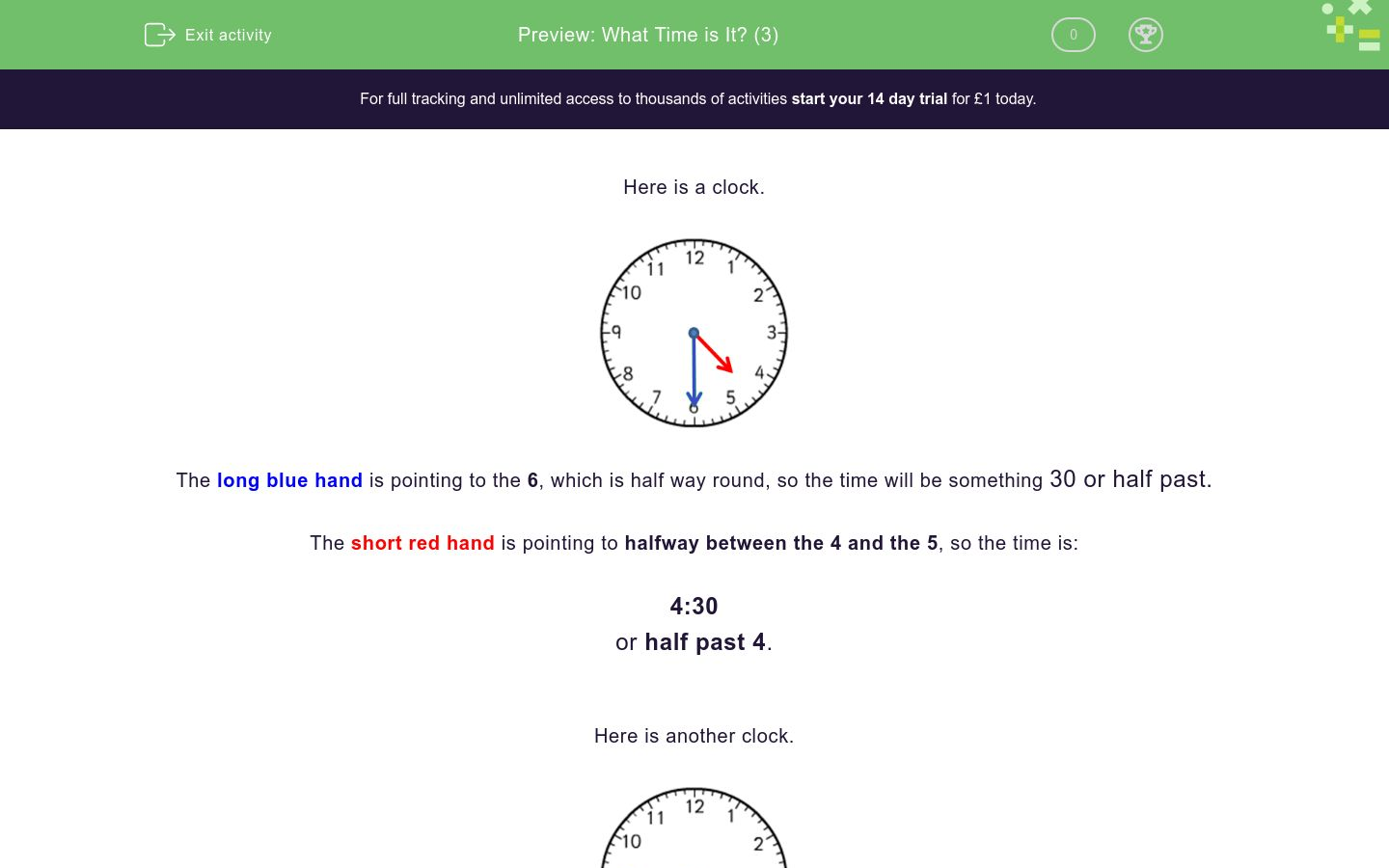Key stage:  KS 1

Curriculum topic:   Measurement

Curriculum subtopic:   Tell/Write the Time

Difficulty level:### QUESTION 1 of 10

Here is a clock.The long blue hand is pointing to the 6, which is half way round, so the time will be something 30 or half past.

The short red hand is pointing to halfway between the 4 and the 5, so the time is:

4:30

or half past 4.

Here is another clock.The long blue hand is pointing to the 9, which is three-quarters the way round, so the time will be something 45 or a quarter to the next hour.

The short red hand is pointing to between the 3 and the 4, so the time is:

3:45

or a quarter to 4.

Here is another clock.Count the minutes in 5s as you go around until you reach the long blue hand.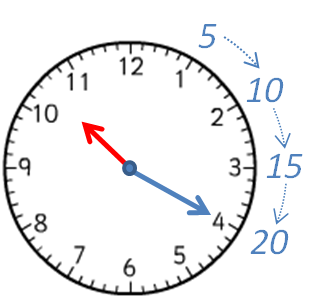The long blue hand is pointing to the 4, which is 4 × 5 = 20 minutes, so the time will be something  20.

The short red hand is pointing to between the 10 and the 11, so the time is:

10:20

or 20 past 10 because it is 20 minutes past 10 o'clock.

Here is another clock.Count the minutes in 5s as you go around until you reach the long blue hand.The long blue hand is pointing to the 7, which is 7 × 5 = 35 minutes, so the time will be something  35.

The short red hand is pointing to between the 2 and the 3, so the time is:

2:35

or 25 to 3 because there are 25 minutes until 3 o'clock.

The time is

5:30 p.m.

Which clock face shows this time?A B C
A

B

C

The time is

2:15 p.m.

Which clock face shows this time?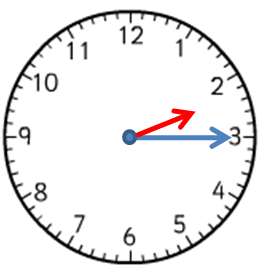A B C
A

B

C

The time is

a quarter to 10.

Which clock face shows this time?A B C
A

B

C

The time is

4:55 p.m.

Which clock face shows this time?A B C
A

B

C

The time is

a quarter past 6.

Which clock face shows this time?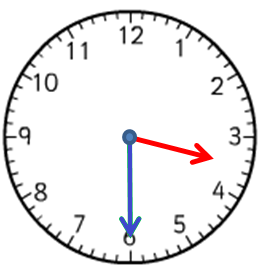A B C
A

B

C

The time is

20 to 8.

Which clock face shows this time?A B C
A

B

C

The time is

3:10 a.m.

Which clock face shows this time?A B C
A

B

C

The time is

9:30 p.m.

Which clock face shows this time?A B C
A

B

C

The time is

half past 6.

Which clock face shows this time?A B C
A

B

C

The time is

5 to 8.

Which clock face shows this time?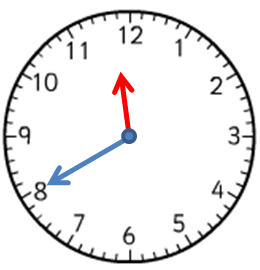A B C
A

B

C

• Question 1

The time is

5:30 p.m.

Which clock face shows this time?A B C
C
EDDIE SAYS
The short hand is pointing to halfway between the 5 and the 6.
The long hand is pointing to 6, which is 30 minutes.
• Question 2

The time is

2:15 p.m.

Which clock face shows this time?A B C
A
EDDIE SAYS
The short hand is pointing to between the 2 and the 3.
The long hand is pointing to 3, which is 15 minutes.
• Question 3

The time is

a quarter to 10.

Which clock face shows this time?A B C
C
EDDIE SAYS
The short hand is pointing to between the 9 and the 10.
The long hand is pointing to 9, which is 45 minutes or 'a quarter to'.
• Question 4

The time is

4:55 p.m.

Which clock face shows this time?A B C
A
EDDIE SAYS
The short hand is pointing to between the 4 and the 5.
The long hand is pointing to 11, which is 55 minutes.
• Question 5

The time is

a quarter past 6.

Which clock face shows this time?A B C
A
EDDIE SAYS
The short hand is pointing to between the 6 and the 7.
The long hand is pointing to 3, which is 'a quarter past' or 15 minutes.
• Question 6

The time is

20 to 8.

Which clock face shows this time?A B C
C
EDDIE SAYS
The short hand is pointing to between the 7 and the 8.
The long hand is pointing to 8, which is 40 minutes or '20 to'.
• Question 7

The time is

3:10 a.m.

Which clock face shows this time?A B C
C
EDDIE SAYS
The short hand is pointing to between the 3 and the 4.
The long hand is pointing to 2, which is 10 minutes.
• Question 8

The time is

9:30 p.m.

Which clock face shows this time?A B C
A
EDDIE SAYS
The short hand is pointing to halfway between the 9 and the 10.
The long hand is pointing to 6, which is 30 minutes.
• Question 9

The time is

half past 6.

Which clock face shows this time?A B C
B
EDDIE SAYS
The short hand is pointing to halfway between the 6 and the 7.
The long hand is pointing to 6, which is 30 minutes.
• Question 10

The time is

5 to 8.

Which clock face shows this time?A B C
C
EDDIE SAYS
The short hand is pointing to between the 7 and the 8.
The long hand is pointing to 11, which is 55 minutes or '5 to'.
---- OR ----

Sign up for a £1 trial so you can track and measure your child's progress on this activity.

### What is EdPlace?

We're your National Curriculum aligned online education content provider helping each child succeed in English, maths and science from year 1 to GCSE. With an EdPlace account you’ll be able to track and measure progress, helping each child achieve their best. We build confidence and attainment by personalising each child’s learning at a level that suits them.

Get started